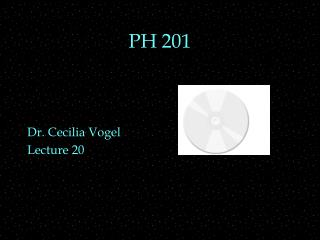DownloadDownload PresentationPH 201

# PH 201

Télécharger la présentation## PH 201

- - - - - - - - - - - - - - - - - - - - - - - - - - - E N D - - - - - - - - - - - - - - - - - - - - - - - - - - -
##### Presentation Transcript

1. PH 201 Dr. Cecilia Vogel Lecture 20

2. REVIEW • Constant angular acceleration equations • Rotational Motion • torque OUTLINE • moment of inertia • angular momentum • angular kinetic energy

3. Table so Far

4. Recall Momentum • Momentum is conserved, • if no external force • because • SF=mDvCM/Dt • So if LHS=0, DvCM=0 • then Dp=0

5. Angular Momentum • St=IDw/Dt • So if LHS =0 • then IDw = 0 • Define angular momentum • Angular momentum conserved • if no net external torque

6. Linear variable Angular variable Variable name x q angle (rad) v = dx/dt w = dq/dt angular velocity (rad/s) a = dv/dt a = dw/dt ang. acceleration (rad/s2) F t torque (Nm) m I moment of inertia (kgm2) K = ½mv2 Krot = ½Iw2 Rotational Kinetic Energy (J) p Add to Table Angular momentum (kgm2/s) L=Iw

7. Angular Momentum • St=IDw/Dt • So if net torque is not zero • then L changes • angular momentum changes

8. Angular Momentum • angular momentum is a vector • direction is found by a RHR • Hold your right hand so your curved fingers point in the direction of rotation • then your thumb will point in the direction of angular momentum (out +, in -)

9. Conservation Demo • Sit on a chair, free to rotate • hold a wheel rotating so its angular momentum points to your left. • Try to tip wheel’s axis up or down. • Notice • torque required for you to change angular momentum of wheel (just direction). • You and wheel are isolated, so if you tip wheel axis down, • to conserve momentum need L ___.

10. Demo and Bikes • Sit on a bike • wheels rotate so angular momentum points to your left. • Lean the bike. • If you tip wheel axis down, (lean left) • to conserve momentum need L ___ • Bike turns ___

11. Kinetic Energy of Rotation • As a rigid body rotates, • all parts are moving • but different parts are moving at different speeds, • so • If you consider • then